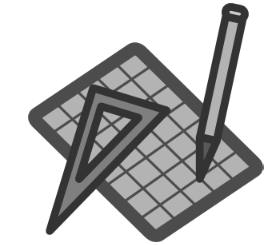## Saturday, 21 July 2012

### CBSE Class 9 - Maths - Ch6 - Lines and AnglesUnderstanding Basic Terms.
Fill in the Blanks

1. A point, a line and a plane are' basic concepts of geometry. These terms are _____ (well defined/undefined).

2. A line with two end points is called a _________ (line-segment/ ray).

3. A terminated line can be produced ________ (within defined limits/indefinitely).

4. A part of a line with one end point is called a _____ (line-segment/ray).

5. A line contains ______ (definite/infinite) number of  points.

6. (One and only one/More than one) _______  line passes through two given points.

7. If three or more points lie on the same line, they are called _________ (collinear / non-collinear) points.

8. Two distinct lines _______ (cannot have/ can have) more than one point in common.

9. A plane contains ______ (definite/infinite) points.

10. A plane contains ______ (definite/infinite) lines.

11. Two rays A and B and having the common initial point A is said to form ____ at A. The point A is called ____ of the angle.

12. The rays making an angle are called the ____ of the angle.

13. If the lines do not lie in the same plane. then they are called ____ (coplaner/ non-coplaner) lines.

14. If three or more lines pass through a point, then these lines are ______ (concurrent/serial/non-concurrent)

15. Straight angle = ____ (0°/90°/180°/360°)

16. Obtuse angle (y) = _____ (90° <  y < 180° or 0° < y < 90°)

17. reflex angle = _____ ( 0° <  y < 90° or 90° <  t < 180° or 180° < t < 360° )

18. Two angles whose sum is 90° are called _______ (complementary/ supplementary)  angles.

19. Two angles whose sum is 180° are called _______ (complementary/ supplementary)  angles.

20. (Intersecting/Parallel) _______ lines are always coplanar.

21. The distance between the end points of a line segment is called the ______ (length/breadth) of the segment.

22. If a ray stands on a line, then the sum of two adjacent angles so formed is ____ (0° /90° /180° / 360°).

23. Two congruent line segments are ____ (equal/unequal) in length.

24. If two lines intersect, then the vertically opposite angles are _______ (equal/unequal).

25. If a transversal intersects two parallel lines, then any pair of interior angles are ________ (complementary/ supplementary).

26. If seg AB ≅ seg AB, i.e. every segment is congruent to itself. This congruent property is called______ (reflexivity/ symmetry/ transitivity).

27. If  seg AB ≅ seg CD, then seg CD ≅ seg AB. This congruent property is called ______ (reflexivity/ symmetry/ transitivity)

28. Two lines in a plane can be ______ (only intersecting/ only parallel/ both intersecting or parallel) lines.

1. undefined.
2. line-segment
3. indefinitely
4. ray
5. infinite
6. One and only one
7. collinear points
8. cannot have
9. infinite
10. infinite
11. angle, vertex
12. arms
13. non-coplaner
14. concurrent
15. 180°
16. 90° <  y < 180°
17. 180° < t < 360°
18. complementary
19. supplementary
20. Parallel
21. length
22. 180°
23. equal
24. equal
25. supplementary
26. reflexivity
27. symmetry
28. both intersecting or parallel1.1.